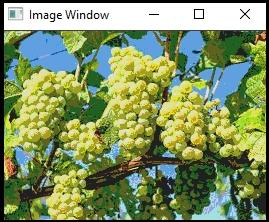# How to reduce color using Pointer Method in OpenCV?

In image processing, we perform computation on the image. To be more specific, we perform a calculation on pixel so the higher the number of pixels, the more the time consuming the computation becomes. To reduce the computation time, we require to scan the image efficiently. We will learn how to implement an efficient image scanning loop using pointers.

Here we will see the pixel scanning process with an example of the color reduction strategy. Color images such as RGB images are composed of 3 channels. Each of these channels has the same number of pixels but with corresponding values. Each of this value is an 8-bit unsigned char value.

Hence, the total number of possible color is 256 x 256 x 256 = 16,777,216. We can divide each pixel's value by cube of equal size to reduce this huge number of possible color. If we divide the values using 8 x 8 x 8 cube then the number of possible color becomes 32 x 32 x 32 = 32,768 colors.

When the number of colors gets reduced, the system becomes fast. To perform this reduction, we need to scan each pixel, which is a time-consuming task. That's why we need an efficient image scanning method.

The following program demonstrates how to reduce the color using the pointer method in OpenCV.

## Example

#include<iostream>
#include<opencv2/highgui/highgui.hpp>
using namespace cv;//Declaring cv namespace
using namespace std;//Declaring std namespace
void reducing_Color(Mat &image, int div=64){ //Declaring the function//
int   total_rows = image.rows;//getting the number of lines//
int total_columns = image.cols * image.channels();//getting the number of columns per line//
for (int j = 0; j < total_rows; j++){ //initiating a for loop for rows
uchar* data = image.ptr<uchar>(j);
for (int i = 0; i < total_columns; i++){ //initiating a for loop for columns//
data[i] = data[i] / div * div + div / 2;//processing the pixels//
}
}
}
int main() {
Mat image;//taking an image matrix//
}